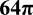# Diagonal of a Cube

Back to “Geometry” Page

The diagonal of a cube is nearly as simple as the Pythagorean theorem.

It is very simple:

(length)2 + (width)2 + (height)2 = (diagonal)2

In other words, you simply take the square root of the sum of the squares of the length, width, and height of the cube.

(length)2 + (width)2 + (height)2   = dA simple equation for the diagram above would be this:

x2 + y2 + z2   = d

Since the length, width, and height of a cube are equal, you can simply multiply one side squared by 3, like this:

3(x2) = d

So, if a side of the cube above is equal to 1, then the equation for the diagonal is this:

3(12) = d

3  = d

It is handy to remember that  3  is approximately 1.7 or so. Just keep that tucked away in your memory.

And, if a side of the cube above is equal to 2, then the equation for the diagonal is this:

3(22) = d

3(4)  = d

2 3  = d

And, if a side of the cube above is equal to 3, then the equation for the diagonal is this:

3(32) = d

3(9)  = d

3 3  = d

You can now see a pattern developing. The diagonal of a cube is equal to any side of the cube times  3 .

See whether you can solve the flashcards below.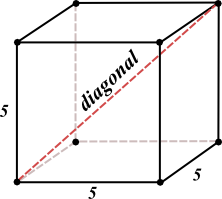• What is the diagonal the cube above?• What is the area of one face of the cube?
52 or 25
• What is the surface area of the cube?
6(25) or 150
• What is the volume of the cube?
53 or 125• What is the diagonal of the cube above?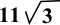• What is the area of one face of the cube?
112 or 121
• What is the surface area of the cube?
6(121) or 726
• What is the volume of the cube?
113 or 1,331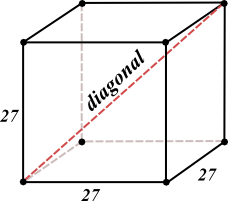• What is the diagonal of the cube above?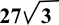• What is the area of one face of the cube?
272 or 729
• What is the surface area of the cube?
6(729) or 4,374
• What is the volume of the cube?
273 or 19,683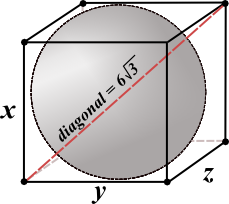Assume that the sphere touches all six sides of the cube in the diagram above.

• What's the diameter of the sphere?
6
• What is the area of one face of the cube?
62 or 36
• What is the surface area of the cube?
6(36) or 216
• What is the volume of the cube?
63 or 216

WAIT! WAIT! Not done yet!
Recall the formula for volume of a sphere:

V = 4/3 π r3

And the formula for surface area of a sphere:

A = 4π r2

• What's the volume of the sphere?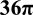• What's the surface area of the sphere?Now, try one more diagram. Remember that standardized tests often give answer choices with fractions, roots and π symbols in the answer.Assume that the sphere touches all six sides of the cube in the diagram above.

• What's the diameter of the sphere?
8
• What is the area of one face of the cube?
82 or 64
• What is the surface area of the cube?
6(64) or 384
• What is the volume of the cube?
83 or 512
• What's the volume of the sphere?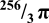• What's the surface area of the sphere?